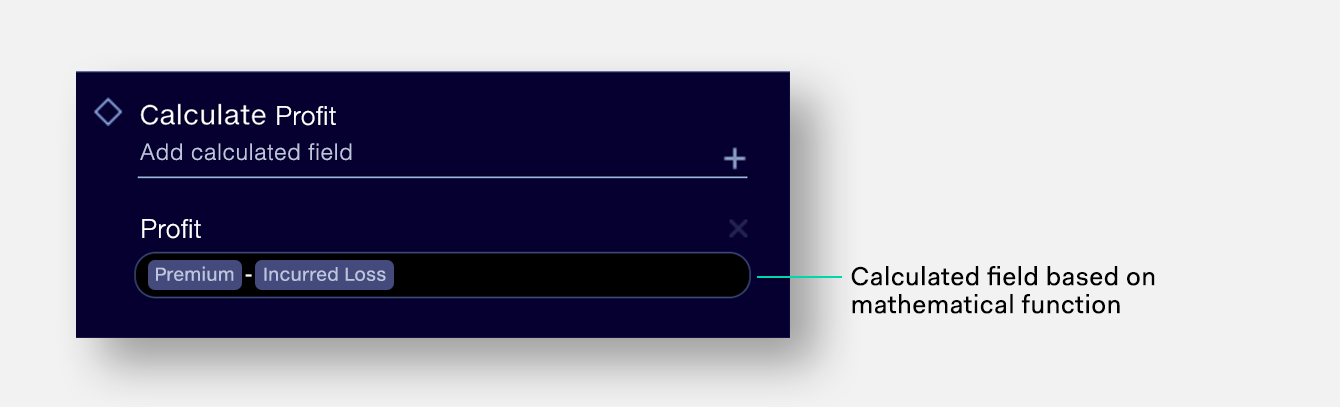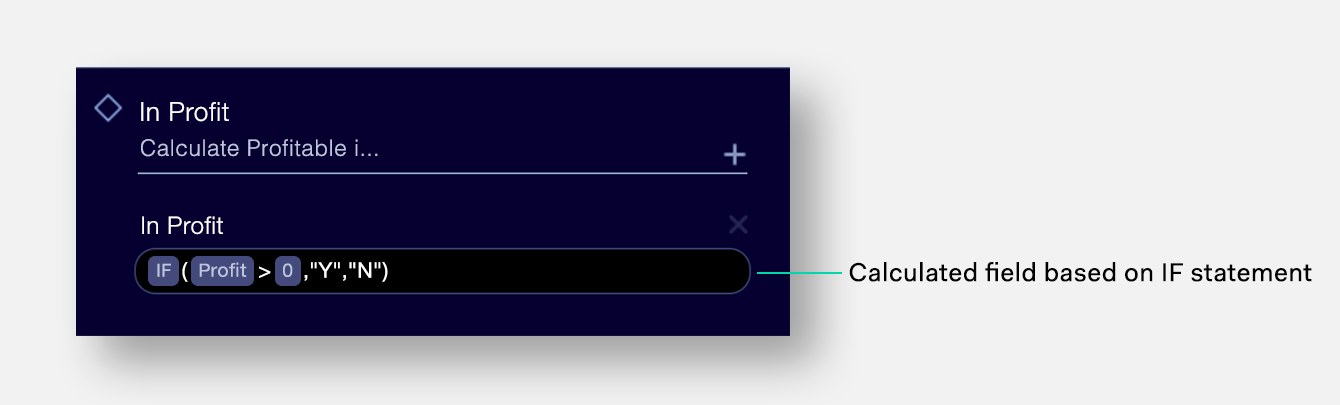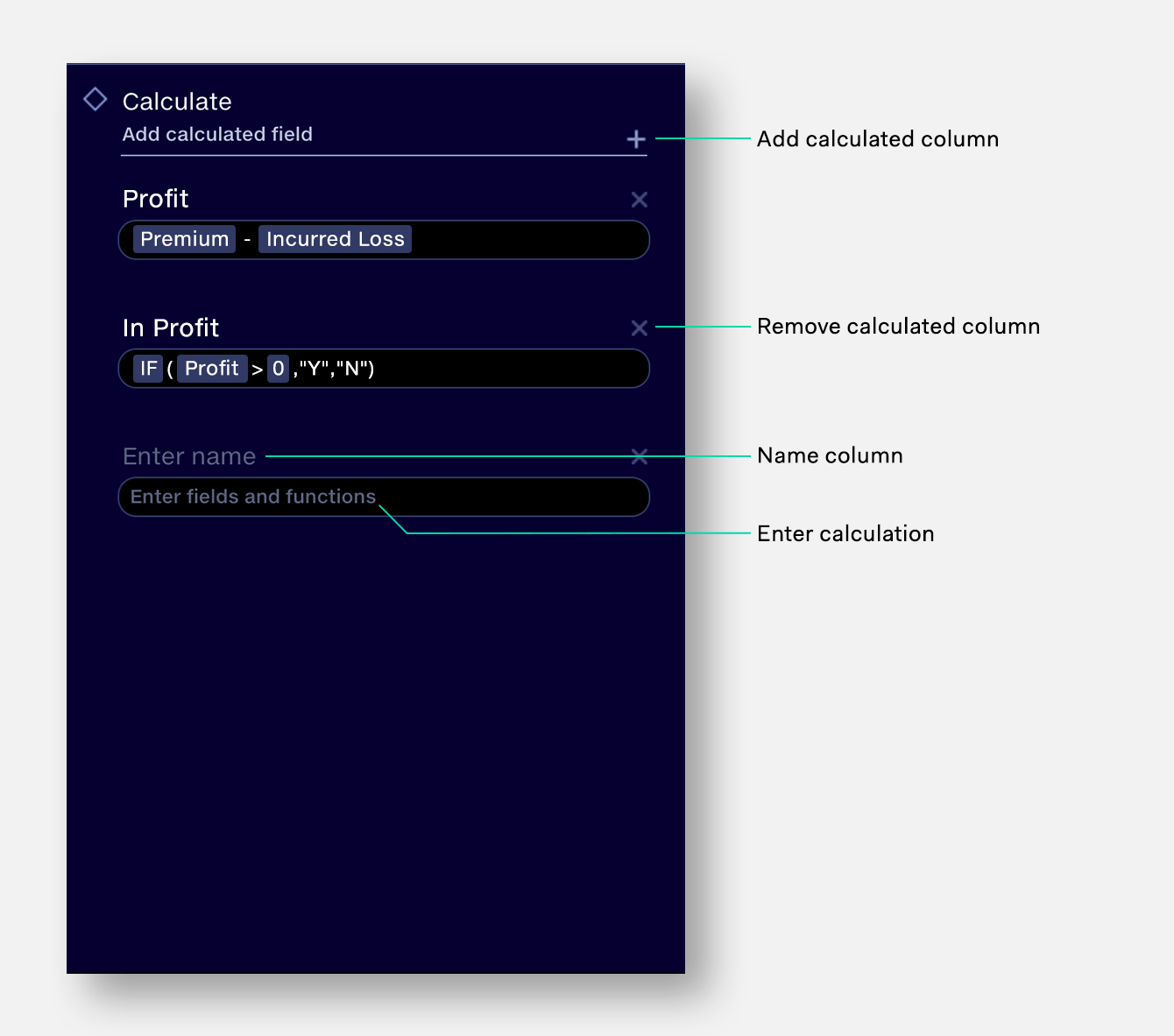# Calculate

The Calculate operation creates new columns of data calculated from your source data. For example, from a column of Premium and a column of Incurred Losses it is possible to calculate a new column
of Profit.The new column is appended to the right side of the output data.

In addition to a full set of arithmetic operators, the Calculate operation utilises a library of advanced functions, such as IF statements. For example, to check whether a policy is profitable create a new column called ‘In Profit’; if the value in the ‘Profit’ column is greater than 0 write ‘Y’, and if not, write ‘N’.See the Syntax and functions section for more details.

Multiple calculated columns can be added within a single Calculate operation, up to a maximum of 51.To create a calculated column:

1. Add a calculate operation from the enrich section in the operations palette.
2. Name the calculated column.
3. Type in the equation field and select from the suggested column names and functions.
4. Run the pipeline to create the calculated column in your output datasets.

### Column ordering and referencing

Calculated columns are added to the right end of the dataset in the order shown in the panel, from top to bottom, and can be reordered via drag and drop.

Calculated columns can reference other calculated columns higher in the list.

The example below demonstrates adding calculated columns, referencing other calculated columns and reordering them.

Note that the column referencing will fail when:

• the referenced columns are renamed.
• the referenced columns are moved below the column you are creating. This is because the columns are created in order, so when the pipeline is run the referenced column will not yet exist to be referenced.

### Bulk-copy calculated columns

Calculated columns can be copied and pasted all at once to another Calculate operation. This is useful for duplicating a set of calculated columns across multiple pipelines.

To copy columns: open a Calculate operation and with no fields selected click ctrl+c (Win) or cmd+c (Mac).

To paste columns: open a Calculate operation and click ctrl+v (Win) or cmd+v (Mac). This will add the copied columns below any existing columns.

To duplicate a Calculate operation: copy the columns from one operation, create a new Calculate operation and paste them in.

Note that the Calculate operation may need the same input column names for the calculations to work.

If the number of pasted columns will cause the total number of columns to to exceed 51, the excess columns will not be pasted.

## Functions index

The Equation Editor in the Calculate and Validate operations supports a range of Excel-like functions for general purpose data cleansing and set of functions specifically for cleansing dates. To define columns, values and operators in functions, use the syntax described in the Operators and syntax section.

## General Functions

### ABS

Returns the absolute value of a number.

``ABS(<column>)``

#### Example

 VALUE ABS('VALUE') 1 1 -1 1

### CONCATENATE

Joins multiple values together to create a single text value.

Note that if named columns are not present, the run will fail. If this is not desired behviour, consider using the Combine Columns operation instead.

``CONCATENATE(<column name or value> … )``

#### Example

 ID CONCATENATE("X", 'ID') 123 X123 1234 X1234

### FIND

Finds a text value and returns position of its first character.

``FIND(<search within>, <search for>)``

Note:

• The first character in the searched text is counted as position 1.
• If no match is found, the value 0 will be returned.
• Searching for a blank value will return 0.
• The match is case-sensitive. To perform a case-insensitive match combine with LOWER, UPPER or PROPER functions:
``FIND(UPPER(<search within>), UPPER(<search for>))``

#### Examples

Find the position of the character ‘d’ within the ID column.

 ID FIND('ID’, “d”) abcd 4 defg 1 efgh 0

Extract the first word of a text value, for example extracting a first name from a full name. Note that in this example, if the name does not contain a space, a blank will be returned.

 NAME LEFT('NAME', FIND('NAME', " ") - 1) Ronald Davies Ronald Jane Alice Smith Jane F. Scott Fitzgerald F. Madonna

Check whether a column contains a given text value, for example check whether a post code contains SE4.

 Postcode IF(FIND('Postcode', "SE4") > 0, "Yes", "No") SE4 5BX Yes SE13 4WZ No SE47RS Yes

Remove the first word, for example remove a building number from a street address. This expression looks for the position of the first space and returns everything after that space, returning ‘Unknown’ if no space is found.

 Street IF(FIND('Street', " ") = 0, "Unknown", MID('Street', FIND('Street', " ") + 1, LENGTH('Street'))) 23 Acacia Avenue Acacia Avenue 361–364 Park Road Park Road London Unknown

### IF

Checks whether a condition is met and returns one value if true, and another value if false.

``IF(<logical query>, <value if true>, <value if false>)``

Examples of logical queries:

``````'Line_of_Business' = "Property"
'Region' = "Americas"
'Claim' > 1000000``````

#### IF statement example

If the Line of Business is Property, then multiply premium by 1.2. If it is not Property leave the Premium value unchanged.

``IF('Line_of_Business' = "Property", 'Premium' * 1.2, 'Premium')``

#### Using and/or in IF statements

And statement

``````IF('Column 1' > 'Column 2' and 'Column 3' < 'Column 4',
value_if_true, value_if_false)``````

Or statement

``````IF('Column 1' > 'Column 2' or 'Column 3' < 'Column 4',
value_if_true, value_if_false)``````

Combined statement

``````IF(('Column 1' > 'Column 2' or 'Column 1' < 'Column 5')
and ('Column 3' < 'Column 4'), value_if_true, value_if_false)``````

### IFERROR

Returns a value if a calculation generates an error.

``IFERROR(<calculation>, <value>)``

#### Example

If the calculation ‘claim_amount / claim_count’ generates an error, return the value 0.

``IFERROR('claim_amount' / 'claim_count', 0)``

### ISNUMBER

Returns TRUE if value is a number, and FALSE if not (for use with IF statements).

``ISNUMBER(<column>)``

#### Example

 value IF(ISNUMBER('value'), "Number", "Not a number") 1 Number "1" Number 1.2 Number "" Not a number "text" Not a number

### ISLIKE

Returns TRUE if value matches a regular expression, and FALSE if not (for use with IF statements)

``ISLIKE(<column>, "regular expression")``

#### Example

 ID IF(ISLIKE('ID', "4\$"), "Ends with 4", "Doesn't end with 4") 123 Doesn’t end with 4 1234 Ends with 4

### LEFT

Returns the first characters from a value, given the number of characters

``LEFT(<column>, <no. of characters>)``

#### Example

 Address LEFT('SIC Code', 4) 0800 Forestry 0800 2080 Beverages 2080

### LENGTH

Returns the length of each column value.

``LENGTH(<column>)``

#### Example

 ID LENGTH('ID') 123 3 1234 4

### LOWER

Converts text to lowercase.

``LOWER(<column name or text value> … )``

### MAX

Returns the maximum value across multiple columns or constant values.

Each parameter can be a numeric value or a column name. If the parameter is a column name then the function will get the value in that column. All the values are compared and the largest value is returned.

``MAX(<column name or numeric value>, … )``

#### Example

 VALUE MAX('VALUE',0) 1 1 -1 0

### MID

Returns characters from a value, given starting position (where first character is 1) and number of characters.

``MID(<column>, <start position>, <no. of characters>)``

#### Example

 ID MID('ID', 4, 2) 12345678 45 abcdefghijkl de

### MIN

Returns the minimum value across multiple columns or constant values.

Each parameter can be a numeric value or a column name. If the parameter is a column name then the function will get the value in that column. All the values are compared and the smallest value is returned.

``MIN(<column name or numeric value>, … )``

#### Example

 VALUE MIN('VALUE',0) 1 0 -1 -1

### PROPER

Converts the first letter of each word to uppercase and the other letters to lowercase.

``PROPER(<column name or text value> … )``

#### Example

 VALUE PROPER('VALUE') SAN FRANCISCO San Francisco sao paulo Sao Paulo SAN DIEGO, CA San Diego, Ca

Returns the last characters from a value, given the number of characters

``RIGHT(<column>, <no. of characters>)``

#### Example

 Address RIGHT('Address', 8) London, EC1Y 1AA EC1Y 1AA Penzance, TR19 7AA TR19 7AA

### ROUND

Rounds a number to a given number of digits.

``ROUND(<column name or numeric value>, <no. of digits>)``

Numbers 1–4 are rounded down and 5–9 are rounded up. Numbers are rounded to a specified level of precision. They can be rounded to the right or left of the decimal point.

If num_digits > 0, number is rounded to the specified number of decimal places to the right of the decimal point.

If num_digits < 0, number is rounded to the left of the decimal point (i.e. to the nearest 10, 100, 1000, etc.).

If num_digits = 0, number is rounded to the nearest integer.

#### Examples

 Formula Description Result ROUND(3.14, 1) Rounds 3.14 to one decimal place 3.1 ROUND(12.34, -1) Rounds 12.34 to one decimal place to the left of the decimal point 10 ROUND(876.5, -3) Rounds 876.5 to the nearest 1000 1000

### SUBSTITUTE

Finds a text value and replaces it with another text value.

``SUBSTITUTE(<search within>, <search for>, <replace with>)``

Note:

• All instances of the searched for text in the searched within text will be replaced.
• If no searched for text is found, the searched within text is not changed.
• If the searched within text is blank, the result will be blank.
• If the searched for text is blank, the result will be the searched within text, unchanged.
• If the replace with text is blank then the searched for text will be removed.
• The match is case-sensitive.

#### Example

Remove dashes from an ID value.

 ID SUBSTITUTE('ID', "-", "") QUAN-1234-785 QUAN1234785 QUAN7436528 QUAN7436528

### SUBSTRING

Returns characters from a value, given starting position (where first character is 0) and number of characters.

``SUBSTRING(<column>, <start position>, <no. of characters>)``

#### Example

 ID SUBSTRING('ID', 4, 2) 12345678 56 abcdefghijkl ef

### TRIM

Removes extra spaces from text: leading and trailing whitespace and repeated spaces between words.

``TRIM(<column name or text value> … )``

### UPPER

Converts text to uppercase.

``UPPER(<column name or text value> … )``

## Date Functions

Date functions operate on cleansed date values which are in Basic ISO format

yyyymmdd
.

• Blank date values are left blank
• Date values that are not in Basic ISO format will cause the pipeline run to fail.

### DATECALC

Returns a new basic-ISO formatted date by adding or removing a term (constant value or column reference) from a basic-ISO date.

``DATECALC(<iso date>, <term (numeric value)>, <unit>)``

• The term is rounded to the lowest whole number (negative values are rounded up towards zero).
• Adding or subtracting by month/year to a day that is invalid (e.g. to 31st April) will truncate to the last valid day of that month
• <unit> is one of "d"/"day"/"days"; "m"/"month"/"months"; or "y"/"year"/"years"

#### Example

 Premium DATECALC('Premium', -1, "year") DATECALC('Premium', 6, "months") 20120629 20110629 20121229 20200229 20190228 20200829 "" "" "" 2012-06-29 (pipeline error) (pipeline error)

### DURATION

Returns the number of whole elapsed units between two basic-ISO dates.

``DURATION(<start>, <end>, <unit>)``

• The result is rounded to the lowest whole number (negative values are rounded up towards zero).
• If the end is before the start, a negative number will be returned.
• <unit> is one of "d"/"day"/"days"; "m"/"month"/"months"; or "y"/"year"/"years"

#### Example

 Start End DURATION('Start', 'End', "days") 20120629 20120630 1 20120630 20120629 -1 "" "" "" 2012-06-29 2012-06-30 (pipeline error)

### ENDOFQUARTER

Moves a date to the last day in a quarter.

``ENDOFQUARTER(<iso date>)``

### ENDOFMONTH

Moves a date to the last day in a month.

``ENDOFMONTH(<iso date>)``

### ENDOFYEAR

Moves a date to the last day in a year.

``ENDOFYEAR(<iso date>)``

### STARTOFQUARTER

Moves a date to the first day in a quarter.

``STARTOFQUARTER(<iso date>)``

### STARTOFMONTH

Moves a date to the first day in a month.

``STARTOFMONTH(<iso date>)``

### STARTOFYEAR

Moves a date to the first day in a year.

``STARTOFYEAR(<iso date>)``

## Operators and syntax

Valid operators in the equation editor:
+
plus

-
minus

/
divide

*
multiply

^
exponent

=
equals

!=
not equals

>
greater than

<
less than

>=
greater than or equal to

<=
less than or equal to
Standard syntax must be followed for the equation editor to function. Quantemplate automatically inserts correct syntax where possible.
‘ ’

“ ”
IF(...)
,